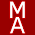## Saturday, September 8, 2012

### Bayes’ Theorem and the Moral Argument

Home  >  Philosophy  >  Atheism/Theism

In my previous blog entry I gave a quick introduction to Bayes’ theorem. In this blog entry I’ll show how Bayes’ theorem can be used in the service of theism.

When I put forth for the moral argument, I that argued that “If God does not exist, then objective morality does not exist” was likely true, and that it seems unlikely on atheism that objective morality exists. Another way to put it: “The probability that objective morality exists given that atheism is true is low.” With the conception of God I’m using, the existence of God entails that objective morality exists (God is morally good independently of human opinion), such that “The probability that objective morality exists given that God exists” is 100%. So we can construct a form of the moral argument that uses Bayes’ theorem and the following symbols:
• H is the hypothesis that God exists.
• ~H is the proposition that God does not exist (and thus that atheism is true).
• E is the evidence of objective morality.
• Pr(E|H) is the probability that objective morality exists given that God exists.
• Pr(E|~H) is the probability that objective morality exists given that atheism is true.
That Pr(E|H) = 1 is pretty straightforward given the conception of God we’re using here, but the other probabilities aren’t so straightforward and will perhaps vary depending on the individual. Still, suppose we have someone who is truly agnostic (and thus Pr(H) and Pr(~H) are both 50%) but comes to believe in objective morality, and assigns the following probabilities:
• Pr(E|~H) = 0.25
• Pr(H) = 0.5
• Pr(~H) = 0.5
• Pr(E|H) = 1
One limitation of Bayes’ theorem is that the probabilities aren’t always clear, e.g. not everyone will agree on the correct value of Pr(E|~H). That said, if we had an agnostic that uses the above probabilities, the rules of probability suggest the agnostic should adjust his probability belief in theism using this version of Bayes’ theorem:

Pr(H|E) =
 Pr(H) × Pr(E|H) Pr(H) × Pr(E|H) + Pr(~H) × Pr(E|~H)

Plugging in the values into Bayes’ theorem...

Pr(H|E) =
 0.5 × 1 0.5 × 1 + 0.5 × 0.25
=
 0.5 0.625
= 0.8

Thus leaving the probability of theism given objective morality at 80%. Your own beliefs about the probabilities of e.g. Pr(E|~H) may differ than those of our hypothetical agnostic. If so, experiment and see what you get!

#### 1 comment:

1.I have a rebuttal to this entry: Bayes’ theorem and the Moral Argument.